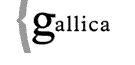Gallica-Math: Répertoire Bibliographique des Sciences Mathématiques (1894-1912)Gallica-Math : Œuvres Complètes | JMPA | RBSM Autres Ressources: Portail Documentaire | NUMDAM | LiNuM

6 articles triés par auteur/année [trier par année/auteur]

Perkins G.-R.  Solution of two similar indeterminate problems. A. [The Analyst] 1, 101-105.
Classification: C1eα Vraies valeurs des expressions indéterminées (cf. D1c ). Fiche 1610
JFM 06.0102.02
Perkins G.-R.  Solution of an indeterminate problem. A. [The Analyst] 1, 151-154.
Classification: C1eα Vraies valeurs des expressions indéterminées (cf. D1c ). Fiche 1610
JFM 06.0109.01
Perkins G.-R.  Solution of two indeterminate problems. A. [The Analyst] 1, 40-43.
Classification: C1eα Vraies valeurs des expressions indéterminées (cf. D1c ). Fiche 1610
Perkins G.-R.  Solution of two problems: 1. Find three square numbers in arithmetical progression, such that if from each its root be substracted, the remainders shall be a squares. — 2. Find three square numbers in arithmetical progression, such that if to each its root be added, the sums shall be squares. A. [The Analyst] 1, 101-105.
Classification: I2 Propriétés générales et élémentaires des nombres. Fiche 1739
JFM 06.0102.02
Perkins G.-R.  Solution of two problems: 1. Find \$n\$ numbers in arithmetical progression, such that the sum of their cubes shall be a square number. — 2. Find \$n\$ consecutive terms, in the natural series of numbers, such that the sum of their cubes shall be a cube number. A. [The Analyst] 1, 40-43.
Classification: I2 Propriétés générales et élémentaires des nombres. Fiche 1739
Perkins G.-R.  Solution of the problem: «Find three right-angled triangles having equal perimeters, and whose areas shall be in arithmetical progression». A. [The Analyst] 1, 151-154.
Classification: K4 Constructions de triangles. Fiche 1794
JFM 06.0109.01